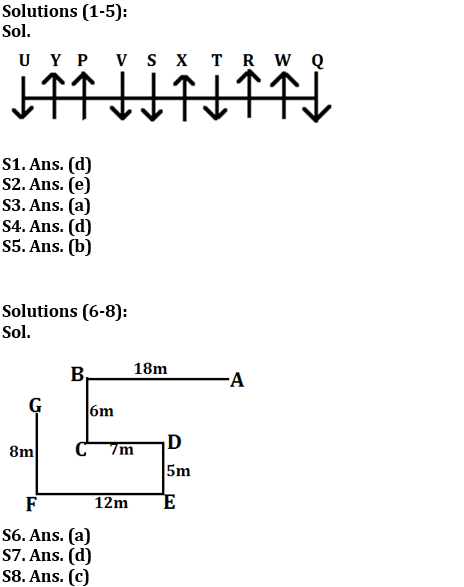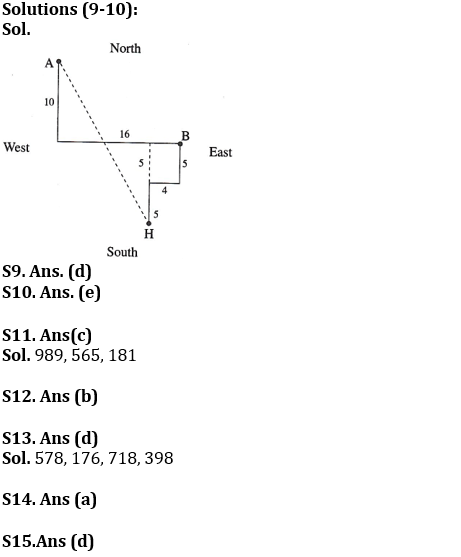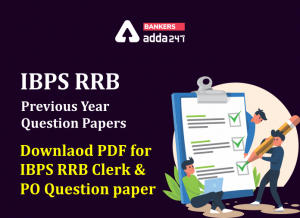Latest Banking jobs   »

# Reasoning Ability Quiz For IBPS RRB PO Clerk Prelims 2022- 9th July

Directions (1-5): Study the information and answer the following questions:
Ten persons sit in a row. Some face north and some face south (but not necessarily in the same manner). Not more than two persons face same direction sits together.
Five persons sit between P and W and both face same direction. Neither P nor W sits at any end. U sits second to the left of P. Q is an immediate neighbor of W. S sits fourth to the left of U and both face same direction. T sits third to the right of Q and faces same direction as Q. Y faces north and is not a neighbor of S and T. X sit second to the left of R and both face same direction. U faces south direction. V neither face same direction as R nor is an immediate neighbor of W.

Q1. Who among the following sits to the immediate right to T?
(a) S
(b) W
(c) U
(d) X
(e) None of these

Q2. How many persons sit between Q and V?
(a) One
(b) Four
(c) Two
(d) Three
(e) More than four

Q3. Four of the following five are alike in a certain way and form a group, who among the following does not belongs to that group?
(a) Q
(b) X
(c) Y
(d) W
(e) P

Q4. Which among the following pair sits at the extreme ends of the row?
(a) P, U
(b) S, X
(c) Q, X
(d) U, Q
(e) None of these

Q5. How many persons face south?
(a) Four
(b) Five
(c) Three
(d) One
(e) Two

Directions (6-8): Study the following information to answer the given questions.
Point B is 18m west of point A. Point G is 8m north of point F. Point E is 5m south of point D which is 7m east of point C. Point F is 12m west of point E. Point C is 6m south of point B.

Q6. What is the direction of point G with respect to point C?
(a) North-west
(b) South-west
(c) North-east
(d) North
(e) None of these

Q7. What is the shortest distance between point F and point D?
(a) 21m
(b) √15m
(c) 10m
(d) 13m
(e) None of these

Q8. What is the direction of point A with respect to point E?
(a) North
(b) East
(c) North-east
(d) South-west
(e) None of these

Directions (9-10): Study the following information to answer the given questions:
A person starts walking in south direction from point A and covers a distance of 10 meters then he takes a left turn and covers a distance of 16 meters and reaches at point B. From point H, another person starts to walk in north direction and covers a distance of 5 meters then after he takes a right turn and covers a distance of 4 meters and finally takes a left turn and covers a distance of 5 meters and reaches at point B.

Q9. Point H is in which direction from Point A?
(a) East
(b) South
(c) North
(d) South-east
(e) South-west

Q10. Point H is in which direction with respect to Point B?
(a) North-West
(b) North
(c) South-East
(d) South
(e) South- West

Directions (11-15): Study the following alphanumeric series carefully and answer the questions given below:

4 3 2 5 6 5 7 8 9 8 9 1 7 6 4 2 3 1 5 7 1 8 1 8 9 7 5 3 9 8 4 3 5

Q11. How many such even numbers are there which is immediately preceded as well as immediately followed by same odd number?
(a) One
(b) Two
(c) Three
(d) Four
(e) None

Q12. Which of the following number is 15th from right end of the series?
(a) 1
(b) 5
(c) 3
(d) 7
(e) None of these

Q13. How many such odd numbers are there which is immediately preceded by odd numbers and immediately followed by even numbers?
(a) Two
(b) None
(c) One
(d) Four
(e) More than four

Q14.How many numbers are there in between the number which is 10th from left end and the number which is 14th from right end?
(a) Nine
(b) Ten
(c) Eleven
(d) Eight
(e) None of these

Q15. If all odd numbers are eliminated from the series, then, which of the following number is 7th from right end of the series?
(a) 8
(b) 4
(c) 2
(d) 6
(e) None of these

Solutions#### Congratulations!Incorrect details? Fill the form again here

•Reasoning Ability Quiz For IBPS RRB PO C...
•Quantitative Aptitude Quiz For IBPS RRB ...
•Reasoning Ability Quiz For IBPS RRB PO C...
•Quantitative Aptitude Quiz For IBPS RRB ...
•IBPS RRB Previous Year Question Paper PD...
•Quantitative Aptitude Quiz For IBPS RRB ...